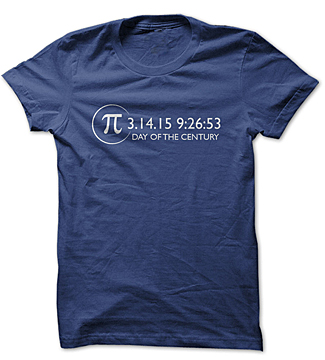## Happy Pi Day 2015

Saturday , 14, March 2015 Comments Off on Happy Pi Day 2015March 14th, 3.14, is celebrated around the world as Pi Day every year. But this year’s Pi Day is even more significant because this year it is 2015 and the first 5 numbers of pi correspond to the format we sometimes give dates; in this case March 14, 2015 is 3.1415.

Pi is a mathematical constant, defined as the ratio of a circle’s circumference to its diameter, commonly approximated as 3.14159, though the actual number is infinitesimal. Here’s how the Dr. Math site describes pi:

Pi is an infinite decimal. Unlike numbers such as 3, 9.876, and 4.5, which have finitely many nonzero numbers to the right of the decimal place, pi has infinitely many numbers to the right of the decimal point.

If you write pi down in decimal form, the numbers to the right of the 0 never repeat in a pattern. Some infinite decimals do have patterns – for instance, the infinite decimal .3333333… has all 3’s to the right of the decimal point, and in the number .123456789123456789123456789… the sequence 123456789 is repeated. However, although many mathematicians have tried to find it, no repeating pattern for pi has been discovered – in fact, in 1768 Johann Lambert proved that there cannot be any such repeating pattern.

As a number that cannot be written as a repeating decimal or a finite decimal (you can never get to the end of it) pi is irrational: it cannot be written as a fraction (the ratio of two integers).An even more detailed Pi Day T-shirt which includes the next 5 numbers, narrowing it down to the hour, minutes and seconds of the day.

And now my brain is mush because math is NOT my strong suit.

But most of us at least know the basics of pi and that the first three numbers correspond to March 14 (and the first 5 numbers correspond to March 14th THIS year) and even we non-mathematicians can enjoy the fun of this particular mathematical expression.

So, Happy Pi Day! May your day be roundly and irrationally enjoyed.

Tags: